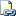# LOG (Transact-SQL)

Returns the natural logarithm of the specified float expression.Transact-SQL Syntax Conventions

## Syntax

``````LOG ( float_expression [, base ] )
``````

## Arguments

• float_expression
Is an expression of type float or of a type that can be implicitly converted to float.

• base
Optional integer argument that sets the base for the logarithm.

float

## Remarks

By default, LOG() returns the natural logarithm. You can change the base of the logarithm to another value using the optional base parameter.

The natural logarithm is the logarithm to the base e, where e is an irrational constant approximately equal to 2.718281828.

The natural logarithm of the exponential of a number is the number itself: LOG( EXP( n ) ) = n. And the exponential of the natural logarithm of a number is the number itself: EXP( LOG( n ) ) = n.

## Examples

### A. Calculating the logarithm for a number.

The following example calculates the LOG for the specified float expression.

``````DECLARE @var float;
SET @var = 10;
SELECT 'The LOG of the variable is: ' + CONVERT(varchar, LOG(@var));
GO
``````

Here is the result set.

``````-------------------------------------
The LOG of the variable is: 2.30259

(1 row(s) affected)
``````

### B. Calculating the logarithm of the exponent of a number.

The following example calculates the LOG for the exponent of a number.

``````SELECT LOG (EXP (10));
``````

Here is the result set.

``````----------------------------------
10
(1 row(s) affected)
``````